# Ball

A setof pointsin a Euclidean spaceat a distance from a given point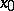(the centre of the ball) less than (an open ball), or not greater than (a closed ball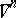) a quantity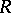(the radius of the ball), i.e.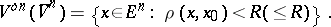A ballis a line segment,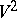is a disc,foris sometimes called a hyperball. The boundary (surface) of a ball is a sphere.

The volume of a ball iswhere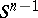is the surface of the boundary sphere andis the gamma-function: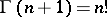,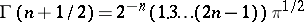. In particular,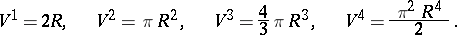With the increase of the dimension, the volume of a ball "concentrates" at its surface:A ball is the simplest geometrical figure. Its topology is trivial. Among all bodies of an equal volume, a ball has minimal surface, and among all bodies of an equal surface, it has maximal volume.

In exactly the same manner a ball can be defined in a metric space; however, in this case it need not be, for example, strictly convex, its surface may have non-smooth points, etc., that is, it may have all phenomena characteristic of arbitrary convex bodies.

Unlike a finite-dimensional ball, an infinite-dimensional ball, being the direct limit of a sequence of balls of successive dimensions imbedded in one another, does not have a compact closure. On the contrary, the compactness of a ball in a topological vector space indicates the finite dimensionality of the latter.

For references, see Sphere.

How to Cite This Entry:
Ball. I.S. Sharadze (originator), Encyclopedia of Mathematics. URL: http://encyclopediaofmath.org/index.php?title=Ball&oldid=16703
This text originally appeared in Encyclopedia of Mathematics - ISBN 1402006098